# AKTU 1st Year Sem 2 Solved Paper 2016-17 | COMP. SYSTEM & C PROGRAMMING | Sec B

• Last Updated : 30 Jan, 2019

# B.Tech.(SEM-II) THEORY EXAMINATION 2016-17COMPUTER SYSTEM & PROGRAMMING IN C

Time: 3hrs
Total Marks: 100

Note:-

• There are three sections. Section A carries 20 marks, Section B carries 30 marks and Section C carries 50 marks.
• Attempt all questions. Marks are indicated against each question.
• Assume suitable data wherever necessary.

# Section – B

2. Attempt any five of the following questions: (5*10 = 50)

1. What is digital computer? Also explain block diagram of digital computer in detail.

Digital Computer: A digital computer can be defined as a programmable machine which reads the binary data passed as instructions, processes this binary data, and displays a calculated digital output. Therefore, Digital computers are those that work on the digital data.

Details of Functional Components of a Digital Computer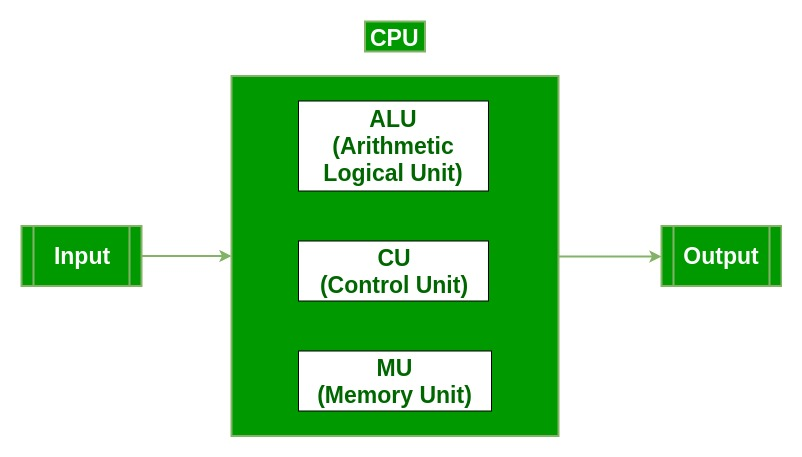• Input Unit :The input unit consists of input devices that are attached to the computer. These devices take input and convert it into binary language that the computer understands. Some of the common input devices are keyboard, mouse, joystick, scanner etc.
• Central Processing Unit (CPU0 : Once the information is entered into the computer by the input device, the processor processes it. The CPU is called the brain of the computer because it is the control center of the computer. It first fetches instructions from memory and then interprets them so as to know what is to be done. If required, data is fetched from memory or input device. Thereafter CPU executes or performs the required computation and then either stores the output or displays on the output device. The CPU has three main components which are responsible for different functions – Arithmetic Logic Unit (ALU), Control Unit (CU) and Memory registers
• Arithmetic and Logic Unit (ALU) : The ALU, as its name suggests performs mathematical calculations and takes logical decisions. Arithmetic calculations include addition, subtraction, multiplication and division. Logical decisions involve comparison of two data items to see which one is larger or smaller or equal.
• Control Unit : The Control unit coordinates and controls the data flow in and out of CPU and also controls all the operations of ALU, memory registers and also input/output units. It is also responsible for carrying out all the instructions stored in the program. It decodes the fetched instruction, interprets it and sends control signals to input/output devices until the required operation is done properly by ALU and memory.
• Memory Registers : A register is a temporary unit of memory in the CPU. These are used to store the data which is directly used by the processor. Registers can be of different sizes(16 bit, 32 bit, 64 bit and so on) and each register inside the CPU has a specific function like storing data, storing an instruction, storing address of a location in memory etc. The user registers can be used by an assembly language programmer for storing operands, intermediate results etc. Accumulator (ACC) is the main register in the ALU and contains one of the operands of an operation to be performed in the ALU.
• Memory : Memory attached to the CPU is used for storage of data and instructions and is called internal memory The internal memory is divided into many storage locations, each of which can store data or instructions. Each memory location is of the same size and has an address. With the help of the address, the computer can read any memory location easily without having to search the entire memory. when a program is executed, it’s data is copied to the internal memory ans is stored in the memory till the end of the execution. The internal memory is also called the Primary memory or Main memory. This memory is also called as RAM, i.e. Random Access Memory. The time of access of data is independent of its location in memory, therefore this memory is also called Random Access memory (RAM). Read this for different types of RAMs
• Output Unit : The output unit consists of output devices that are attached with the computer. It converts the binary data coming from CPU to human understandable form. The common output devices are monitor, printer, plotter etc.
2. Write a program that calculate sum of the digits of an integer. For example, the sum of the digit of the number 2155 is 2+1+5+5 or 13. The program should accept any arbitrary number typed by user.
 `// C program to compute sum of digits in``// number.`` ` `#include `` ` `/* Function to get sum of digits */``int` `getSum(``int` `n)``{``    ``int` `sum = 0;``    ``while` `(n != 0) {``        ``sum = sum + n % 10;``        ``n = n / 10;``    ``}``    ``return` `sum;``}`` ` `// Driver code``int` `main()``{`` ` `    ``int` `n;`` ` `    ``// Get the number``    ``scanf``(``"%d"``, &n);``    ``printf``(``"Enter the number: %d"``, n);`` ` `    ``// Print the digits of the number``    ``printf``(``"\nSum of Digits: %d "``,``           ``getSum(n));`` ` `    ``return` `0;``}`

Output:

```Enter the number: 32764
Sum of Digits: 22 ```
3. Write a program to copy the contents of one array into another in the reverse order.
 `#include `` ` `// Driver code``int` `main()``{``    ``int` `original_arr, copied_arr, i;`` ` `    ``// Get the numbers in the array``    ``printf``(``"\nEnter the Elements: "``);``    ``for` `(i = 0; i < 10; i++) {``        ``scanf``(``"%d"``, &original_arr[i]);``        ``printf``(``"%d, "``, original_arr[i]);``    ``}`` ` `    ``// Copy the elements of the array``    ``// in the copied_arr in Reverse Order``    ``for` `(i = 0; i < 10; i++) {``        ``copied_arr[i] = original_arr[10 - i - 1];``    ``}`` ` `    ``// Print the original_arr``    ``printf``(``"\nOriginal array: "``);``    ``for` `(i = 0; i < 10; i++) {``        ``printf``(``"%d "``, original_arr[i]);``    ``}`` ` `    ``// Print the copied array``    ``printf``(``"\nResultant array: "``);``    ``for` `(i = 0; i < 10; i++) {``        ``printf``(``"%d "``, copied_arr[i]);``    ``}`` ` `    ``return` `0;``}`

Output:

```Enter the Elements: 1, 2, 3, 4, 5, 6, 7, 8, 9, 10,
Original array: 1 2 3 4 5 6 7 8 9 10
Resultant array: 10 9 8 7 6 5 4 3 2 1
```
4. What is Operating System? Also define types and functions of OS.

Operating System – Definition:
• An operating system is a program that controls the execution of application programs and acts as an interface between the user of a computer and the computer hardware.
• A more common definition is that the operating system is the one program running at all times on the computer (usually called the kernel), with all else being application programs.
• An operating system is concerned with the allocation of resources and services, such as memory, processors, devices and information. The operating system correspondingly includes programs to manage these resources, such as a traffic controller, a scheduler, memory management module, I/O programs, and a file system.

Functions of Operating system – Operating system performs three functions:

1. Convenience: An OS makes a computer more convenient to use.
2. Efficiency: An OS allows the computer system resources to be used in an efficient manner.
3. Ability to Evolve: An OS should be constructed in such a way as to permit the effective development, testing and introduction of new system functions without at the same time interfering with service.

Types of Operating System

• Batch Operating System- Sequence of jobs in a program on a computer without manual interventions.
• Time sharing operating System- allows many users to share the computer resources.(Max utilization of the resources).
• Distributed operating System- Manages a group of different computers and make appear to be a single computer.
• Network operating system- computers running in different operating system can participate in common network (It is used for security purpose).
• Real time operating system – meant applications to fix the deadlines.

Examples of Operating System are –

• Windows (GUI based, PC)
• GNU/Linux (Personal, Workstations, ISP, File and print server, Three-tier client/Server)
• macOS (Macintosh), used for Apple’s personal computers and work stations (MacBook, iMac).
• Android (Google’s Operating System for smartphones/tablets/smartwatches)
• iOS (Apple’s OS for iPhone, iPad and iPod Touch)
5. Write a program to multiply two matrices (read size and number of element of matrices from the keyboard).
 `// C program to multiply two square matrices.`` ` `#include `` ` `const` `int` `MAX = 100;`` ` `// Function to print Matrix``void` `printMatrix(``int` `M[][MAX], ``int` `rowSize, ``int` `colSize)``{``    ``for` `(``int` `i = 0; i < rowSize; i++) {``        ``for` `(``int` `j = 0; j < colSize; j++)``            ``printf``(``"%d "``, M[i][j]);`` ` `        ``printf``(``"\n"``);``    ``}``}`` ` `// Function to multiply two matrices A[][] and B[][]``void` `multiplyMatrix(``int` `row1, ``int` `col1, ``int` `A[][MAX],``                    ``int` `row2, ``int` `col2, ``int` `B[][MAX])``{``    ``int` `i, j, k;`` ` `    ``// Matrix to store the result``    ``int` `C[MAX][MAX];`` ` `    ``// Check if multiplication is Possible``    ``if` `(row2 != col1) {``        ``printf``(``"Not Possible\n"``);``        ``return``;``    ``}`` ` `    ``// Multiply the two``    ``for` `(i = 0; i < row1; i++) {``        ``for` `(j = 0; j < col2; j++) {``            ``C[i][j] = 0;``            ``for` `(k = 0; k < row2; k++)``                ``C[i][j] += A[i][k] * B[k][j];``        ``}``    ``}`` ` `    ``// Print the result``    ``printf``(``"\nResultant Matrix: \n"``);``    ``printMatrix(C, row1, col2);``}`` ` `// Driven Program``int` `main()``{``    ``int` `row1, col1, row2, col2, i, j;``    ``int` `A[MAX][MAX], B[MAX][MAX];`` ` `    ``// Read size of Matrix A from user``    ``printf``(``"Enter the number of rows of First Matrix: "``);``    ``scanf``(``"%d"``, &row1);``    ``printf``(``"%d"``, row1);``    ``printf``(``"\nEnter the number of columns of First Matrix: "``);``    ``scanf``(``"%d"``, &col1);``    ``printf``(``"%d"``, col1);`` ` `    ``// Read the elements of Matrix A from user``    ``printf``(``"\nEnter the elements of First Matrix: "``);``    ``for` `(i = 0; i < row1; i++) {``        ``for` `(j = 0; j < col1; j++) {``            ``printf``(``"\nA[%d][%d]: "``, i, j);``            ``scanf``(``"%d"``, &A[i][j]);``            ``printf``(``"%d"``, A[i][j]);``        ``}``    ``}`` ` `    ``// Read size of Matrix B from user``    ``printf``(``"\nEnter the number of rows of Second Matrix: "``);``    ``scanf``(``"%d"``, &row2);``    ``printf``(``"%d"``, row2);``    ``printf``(``"\nEnter the number of columns of Second Matrix: "``);``    ``scanf``(``"%d"``, &col2);``    ``printf``(``"%d"``, col2);`` ` `    ``// Read the elements of Matrix B from user``    ``printf``(``"\nEnter the elements of First Matrix: "``);``    ``for` `(i = 0; i < row2; i++) {``        ``for` `(j = 0; j < col2; j++) {``            ``printf``(``"\nB[%d][%d]: "``, i, j);``            ``scanf``(``"%d"``, &B[i][j]);``            ``printf``(``"%d"``, B[i][j]);``        ``}``    ``}`` ` `    ``// Print the Matrix A``    ``printf``(``"\n\nFirst Matrix: \n"``);``    ``printMatrix(A, row1, col1);`` ` `    ``// Print the Matrix B``    ``printf``(``"\nSecond Matrix: \n"``);``    ``printMatrix(B, row2, col2);`` ` `    ``// Find the product of the 2 matrices``    ``multiplyMatrix(row1, col1, A, row2, col2, B);`` ` `    ``return` `0;``}`
Output:
```Enter the number of rows of First Matrix: 2
Enter the number of columns of First Matrix: 3
Enter the elements of First Matrix:
A: 1
A: 2
A: 3
A: 4
A: 5
A: 6

Enter the number of rows of Second Matrix: 3
Enter the number of columns of Second Matrix: 2
Enter the elements of First Matrix:
B: 1
B: 2
B: 3
B: 4
B: 5
B: 6

First Matrix:
1 2 3
4 5 6

Second Matrix:
1 2
3 4
5 6

Resultant Matrix:
22 28
49 64
```
6. Write a program to sort an integer array in ascending order.
 `// C program for implementation of selection sort``#include `` ` `void` `swap(``int``* xp, ``int``* yp)``{``    ``int` `temp = *xp;``    ``*xp = *yp;``    ``*yp = temp;``}`` ` `void` `selectionSort(``int` `arr[], ``int` `n)``{``    ``int` `i, j, min_idx;`` ` `    ``// One by one move boundary of unsorted subarray``    ``for` `(i = 0; i < n - 1; i++) {``        ``// Find the minimum element in unsorted array``        ``min_idx = i;``        ``for` `(j = i + 1; j < n; j++)``            ``if` `(arr[j] < arr[min_idx])``                ``min_idx = j;`` ` `        ``// Swap the found minimum element with the first element``        ``swap(&arr[min_idx], &arr[i]);``    ``}``}`` ` `/* Function to print an array */``void` `printArray(``int` `arr[], ``int` `size)``{``    ``int` `i;``    ``for` `(i = 0; i < size; i++)``        ``printf``(``"%d "``, arr[i]);``    ``printf``(``"\n"``);``}`` ` `// Driver program to test above functions``int` `main()``{``    ``int` `arr[] = { 64, 25, 12, 22, 11 };``    ``int` `n = ``sizeof``(arr) / ``sizeof``(arr);``    ``selectionSort(arr, n);``    ``printf``(``"Sorted array: \n"``);``    ``printArray(arr, n);``    ``return` `0;``}`

Output:

```Sorted array:
11 12 22 25 64```
7. What is string? Also explain different string functions. Write a user define function to compare two strings where they are identical or not.

Strings are defined as an array of characters. The difference between a character array and a string is the string is terminated with a special character ‘\0’.

Declaring a string is as simple as declaring a one dimensional array. Below is the basic syntax for declaring a string in C programming language.

```char str_name[size];
```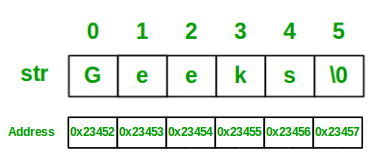Some of the most commonly used String functions are:

• strcat: The strcat() function will append a copy of the source string to the end of destination string.
• strchr: In C/C++, strrchr() is a predefined function used for string handling. cstring is the header file required for string functions.

This function Returns a pointer to the last occurrence of a character in a string.
The character whose last occurrence we want to find in passed as the second argument to the function and the string in which we have to find the character is passed as the first argument to the function.

• strcmp: strcmp() is a built-in library function and is declared in <string.h> header file. This function takes two strings as arguments and compare these two strings lexicographically.
• strcpy: strcpy() is a standard library function in C/C++ and is used to copy one string to another. In C it is present in string.h header file and in C++ it is present in cstring header file.
• strlen: The strlen() function calculates the length of a given string.The strlen() function is defined in string.h header file. It doesn’t count null character ‘\0’.
• strncat: In C/C++, strncat() is a predefined function used for string handling. string.h is the header file required for string functions.

This function appends not more than n characters from the string pointed to by src to the end of the string pointed to by dest plus a terminating Null-character. The initial character of string(src) overwrites the Null-character present at the end of string(dest). Thus, length of the string(dest) becomes strlen(dest)+n. But, if the length of the string(src) is less than n, only the content up to the terminating null-character is copied and length of the string(dest) becomes strlen(src) + strlen(dest).

The behavior is undefined if

• the strings overlap.
• the dest array is not large enough to append the contents of src.
• strncmp: std::strncmp() function lexicographically compares not more than count characters from the two null-terminated strings and returns an integer based on the outcome.
• This function takes two strings and a number num as arguments and compare at most first num bytes of both the strings.
• num should be at most equal to the length of the longest string. If num is defined greater than the string length than comparison is done till the null-character(‘\0’) of either string.
• This function compares the two strings lexicographically. It starts comparison from the first character of each string. If they are equal to each other, it continues and compare the next character of each string and so on.
• This process of comparison stops until a terminating null-character of either string is reached or num characters of both the strings matches.
• strncpy: The strncpy() function is similar to strcpy() function, except that at most n bytes of src are copied. If there is no NULL character among the first n character of src, the string placed in dest will not be NULL-terminated. If the length of src is less than n, strncpy() writes additional NULL character to dest to ensure that a total of n character are written.
• strrchr: The strrchr() function in C/C++ locates the last occurrence of a character in a string. It returns a pointer to the last occurrence in the string. The terminating null character is considered part of the C string. Therefore, it can also be located to retrieve a pointer to the end of a string. It is defined in cstring header file.

Program to check if two strings are identical or not:

 `// C program to check if``// two strings are identical`` ` `#include ``#include `` ` `int` `main()``{`` ` `    ``char` `string1, string2;`` ` `    ``// Get the strings which``    ``// is to be checked``    ``scanf``(``"%s"``, string1);``    ``printf``(``"Enter the first string: %s"``, string1);`` ` `    ``// Get the strings which``    ``// is to be checked``    ``scanf``(``"%s"``, string2);``    ``printf``(``"\nEnter the second string: %s"``, string2);`` ` `    ``// Check if both strings are equal``    ``printf``(``"\nAre both strings same: "``);`` ` `    ``if` `(``strcmp``(string1, string2) == 0) {``        ``printf``(``"Yes"``);``    ``}``    ``else` `{``        ``printf``(``"No"``);``    ``}`` ` `    ``return` `0;``}`

Output:

```Enter the first string: GeeksForGeeks
Enter the second string: GeeksForGeeks
Are both strings same: Yes
```
8. Define the concept of pointer? Also define the dynamic memory allocation and its various functions.

Pointers are symbolic representation of addresses. They enable programs to simulate call-by-reference as well as to create and manipulate dynamic data structures. It’s general declaration in C/C++ has the format:

Syntax:

```datatype *var_name;
int *ptr;   //ptr can point to an address which holds int data
```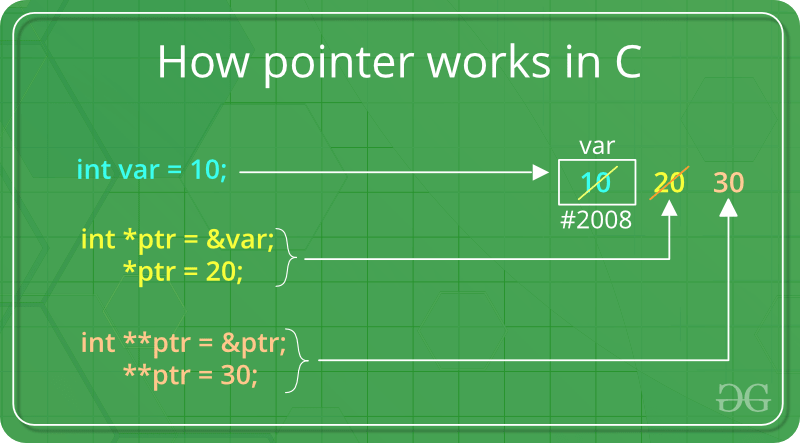How to use a pointer?

• Define a pointer variable
• Assigning the address of a variable to a pointer using unary operator (&) which returns the address of that variable.
• Accessing the value stored in the address using unary operator (*) which returns the value of the variable located at the address specified by its operand.

The reason we associate data type to a pointer is that it knows how many bytes the data is stored in. When we increment a pointer, we increase the pointer by the size of data type to which it points.Dynamic Memory Allocation in C: It can be defined as a procedure in which the size of a data structure (like Array) is changed during the runtime.

C provides some functions to achieve these tasks. There are 4 library functions provided by C defined under <stdlib.h> header file to facilitate dynamic memory allocation in C programming. They are:

1. malloc()
2. calloc()
3. free()
4. realloc()

Lets see each of them in detail.

1. ### malloc()

“malloc” or “memory allocation” method is used to dynamically allocate a single large block of memory with the specified size. It returns a pointer of type void which can be cast into a pointer of any form.

Syntax:

```ptr = (cast-type*) malloc(byte-size)

For Example:
ptr = (int*) malloc(100 * sizeof(int));

Since the size of int is 4 bytes,
this statement will allocate 400 bytes of memory.
And, the pointer ptr holds the address
of the first byte in the allocated memory.
```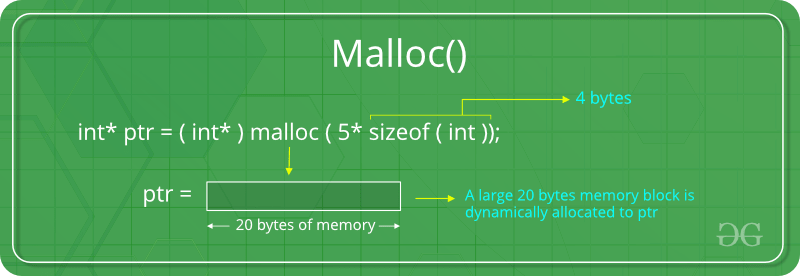If the space is insufficient, allocation fails and returns a NULL pointer.

Example:

 `#include ``#include `` ` `int` `main()``{`` ` `    ``// This pointer will hold the``    ``// base address of the block created``    ``int``* ptr;``    ``int` `n, i, sum = 0;`` ` `    ``// Get the number of elements for the array``    ``n = 5;``    ``printf``(``"Enter number of elements: %d\n"``, n);`` ` `    ``// Dynamically allocate memory using malloc()``    ``ptr = (``int``*)``malloc``(n * ``sizeof``(``int``));`` ` `    ``// Check if the memory has been successfully``    ``// allocated by malloc or not``    ``if` `(ptr == NULL) {``        ``printf``(``"Memory not allocated.\n"``);``        ``exit``(0);``    ``}``    ``else` `{`` ` `        ``// Memory has been successfully allocated``        ``printf``(``"Memory successfully allocated using malloc.\n"``);`` ` `        ``// Get the elements of the array``        ``for` `(i = 0; i < n; ++i) {``            ``ptr[i] = i + 1;``        ``}`` ` `        ``// Print the elements of the array``        ``printf``(``"The elements of the array are: "``);``        ``for` `(i = 0; i < n; ++i) {``            ``printf``(``"%d, "``, ptr[i]);``        ``}``    ``}`` ` `    ``return` `0;``}`
Output:
```Enter number of elements: 5
Memory successfully allocated using malloc.
The elements of the array are: 1, 2, 3, 4, 5,
```
2. ### calloc()

“calloc” or “contiguous allocation” method is used to dynamically allocate the specified number of blocks of memory of the specified type. It initializes each block with a default value ‘0’.

Syntax:

```ptr = (cast-type*)calloc(n, element-size);

For Example:
ptr = (float*) calloc(25, sizeof(float));

This statement allocates contiguous space in memory
for 25 elements each with the size of float.
```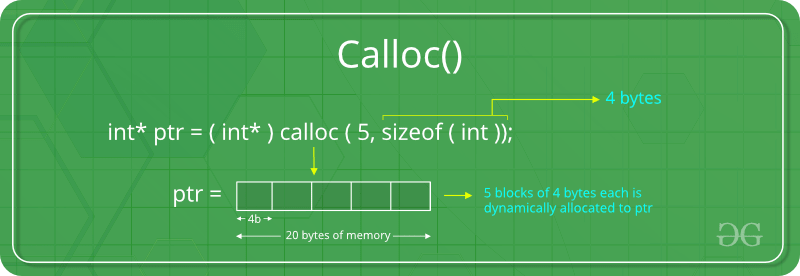If the space is insufficient, allocation fails and returns a NULL pointer.

Example:

 `#include ``#include `` ` `int` `main()``{`` ` `    ``// This pointer will hold the``    ``// base address of the block created``    ``int``* ptr;``    ``int` `n, i, sum = 0;`` ` `    ``// Get the number of elements for the array``    ``n = 5;``    ``printf``(``"Enter number of elements: %d\n"``, n);`` ` `    ``// Dynamically allocate memory using calloc()``    ``ptr = (``int``*)``calloc``(n, ``sizeof``(``int``));`` ` `    ``// Check if the memory has been successfully``    ``// allocated by malloc or not``    ``if` `(ptr == NULL) {``        ``printf``(``"Memory not allocated.\n"``);``        ``exit``(0);``    ``}``    ``else` `{`` ` `        ``// Memory has been successfully allocated``        ``printf``(``"Memory successfully allocated using calloc.\n"``);`` ` `        ``// Get the elements of the array``        ``for` `(i = 0; i < n; ++i) {``            ``ptr[i] = i + 1;``        ``}`` ` `        ``// Print the elements of the array``        ``printf``(``"The elements of the array are: "``);``        ``for` `(i = 0; i < n; ++i) {``            ``printf``(``"%d, "``, ptr[i]);``        ``}``    ``}`` ` `    ``return` `0;``}`
Output:
```Enter number of elements: 5
Memory successfully allocated using calloc.
The elements of the array are: 1, 2, 3, 4, 5,
```
3. ### free()

“free” method is used to dynamically de-allocate the memory. The memory allocated using functions malloc() and calloc() are not de-allocated on their own. Hence the free() method is used, whenever the dynamic memory allocation takes place. It helps to reduce wastage of memory by freeing it.

Syntax:

```free(ptr);
```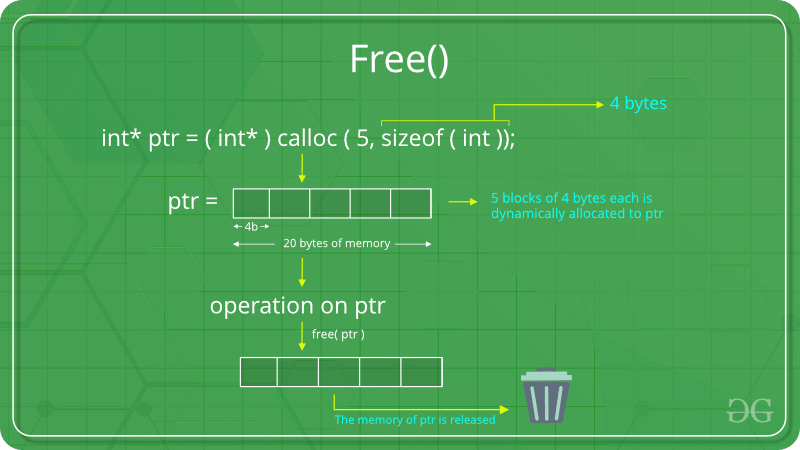Example:

 `#include ``#include `` ` `int` `main()``{`` ` `    ``// This pointer will hold the``    ``// base address of the block created``    ``int` `*ptr, *ptr1;``    ``int` `n, i, sum = 0;`` ` `    ``// Get the number of elements for the array``    ``n = 5;``    ``printf``(``"Enter number of elements: %d\n"``, n);`` ` `    ``// Dynamically allocate memory using malloc()``    ``ptr = (``int``*)``malloc``(n * ``sizeof``(``int``));`` ` `    ``// Dynamically allocate memory using calloc()``    ``ptr1 = (``int``*)``calloc``(n, ``sizeof``(``int``));`` ` `    ``// Check if the memory has been successfully``    ``// allocated by malloc or not``    ``if` `(ptr == NULL || ptr1 == NULL) {``        ``printf``(``"Memory not allocated.\n"``);``        ``exit``(0);``    ``}``    ``else` `{`` ` `        ``// Memory has been successfully allocated``        ``printf``(``"Memory successfully allocated using malloc.\n"``);`` ` `        ``// Free the memory``        ``free``(ptr);``        ``printf``(``"Malloc Memory successfully freed.\n"``);`` ` `        ``// Memory has been successfully allocated``        ``printf``(``"\nMemory successfully allocated using calloc.\n"``);`` ` `        ``// Free the memory``        ``free``(ptr1);``        ``printf``(``"Calloc Memory successfully freed.\n"``);``    ``}`` ` `    ``return` `0;``}`
Output:
```Enter number of elements: 5
Memory successfully allocated using malloc.
Malloc Memory successfully freed.

Memory successfully allocated using calloc.
Calloc Memory successfully freed.
```
4. ### realloc()

“realloc” or “re-allocation” method is used to dynamically change the memory allocation of a previously allocated memory. In other words, if the memory previously allocated with the help of malloc or calloc is insufficient, realloc can be used to dynamically re-allocate memory.

Syntax:

```ptr = realloc(ptr, newSize);

where ptr is reallocated with new size 'newSize'.
```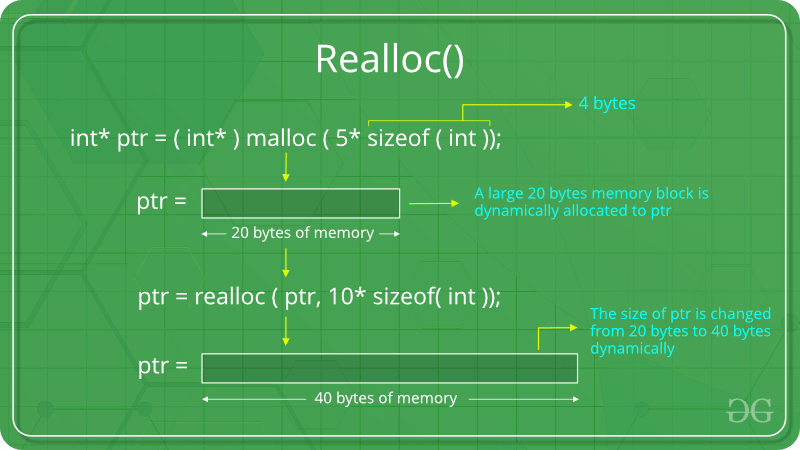If the space is insufficient, allocation fails and returns a NULL pointer.

Example:

 `#include ``#include `` ` `int` `main()``{`` ` `    ``// This pointer will hold the``    ``// base address of the block created``    ``int``* ptr;``    ``int` `n, i, sum = 0;`` ` `    ``// Get the number of elements for the array``    ``n = 5;``    ``printf``(``"Enter number of elements: %d\n"``, n);`` ` `    ``// Dynamically allocate memory using calloc()``    ``ptr = (``int``*)``calloc``(n, ``sizeof``(``int``));`` ` `    ``// Check if the memory has been successfully``    ``// allocated by malloc or not``    ``if` `(ptr == NULL) {``        ``printf``(``"Memory not allocated.\n"``);``        ``exit``(0);``    ``}``    ``else` `{`` ` `        ``// Memory has been successfully allocated``        ``printf``(``"Memory successfully allocated using calloc.\n"``);`` ` `        ``// Get the elements of the array``        ``for` `(i = 0; i < n; ++i) {``            ``ptr[i] = i + 1;``        ``}`` ` `        ``// Print the elements of the array``        ``printf``(``"The elements of the array are: "``);``        ``for` `(i = 0; i < n; ++i) {``            ``printf``(``"%d, "``, ptr[i]);``        ``}`` ` `        ``// Get the new size for the array``        ``n = 10;``        ``printf``(``"\n\nEnter the new size of the array: %d\n"``, n);`` ` `        ``// Dynamically re-allocate memory using realloc()``        ``ptr = ``realloc``(ptr, n * ``sizeof``(``int``));`` ` `        ``// Memory has been successfully allocated``        ``printf``(``"Memory successfully re-allocated using realloc.\n"``);`` ` `        ``// Get the new elements of the array``        ``for` `(i = 5; i < n; ++i) {``            ``ptr[i] = i + 1;``        ``}`` ` `        ``// Print the elements of the array``        ``printf``(``"The elements of the array are: "``);``        ``for` `(i = 0; i < n; ++i) {``            ``printf``(``"%d, "``, ptr[i]);``        ``}`` ` `        ``free``(ptr);``    ``}`` ` `    ``return` `0;``}`
Output:
```Enter number of elements: 5
Memory successfully allocated using calloc.
The elements of the array are: 1, 2, 3, 4, 5,

Enter the new size of the array: 10
Memory successfully re-allocated using realloc.
The elements of the array are: 1, 2, 3, 4, 5, 6, 7, 8, 9, 10,
```

My Personal Notes arrow_drop_up